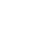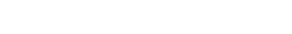# Understanding Percents on the GMAT

Here are five quick tips to make you much more effective at interpreting and solving GMAT problems involving percents, one of the most common GMAT questions.

## Percents and Decimals

Fundamentally, a percent is a fraction out of 100 – it is per centum (Latin for “per 100”).  It’s easy to change a percent to a decimal.  For example, 37% means 37 parts out of one hundred, or 37/100.  As a decimal, that’s just 0.37.  Changing a percent to a decimal simply involves sliding the decimal to the left two places.

## Percent Changes as Multipliers

This is one of the BIG math ideas for GMAT.  A multiplier is a factor by which you multiply a number to get a desired result. There are three percent-related multipliers you will need to understand

a) X% of a number

Suppose I have \$400 in an account, and need to know what 30% of this account is.  The multiplier = the percent as a decimal.  30% as a decimal is 0.30, and \$400(0.30) = \$120, so \$120 is 30% of \$400.

b) an X% increase

Suppose I have \$400 in an account, over time period, I am going to get an additional 5% of interest; in other word, my account is going to increase by 5%.  Here, the multiplier = 1 + (the percent as a decimal).  Thus, \$400(1.05) = \$420, so that’s the amount I would have after a 5% increase

c) an X% decrease

Suppose I have \$400 in an account, and because of some kind of penalty, I am going to be nailed with a 15% deduction; in other words, my account will decrease by 15%.  Here, the multiplier = 1 – (the percent as a decimal).  In this case, the multiplier = 1 – 0.15 = 0.85, and the result after the deduction is (\$400)(0.85) = \$340.

## Calculating a percent change

Basically, a percent is a simpleratio times 100.  The GMAT will ask you to calculate percent changes, and here you have to be very careful with order, i.e., what’s the starting number and what’s the ending number.  IMPORTANT: in a percent change, the starting number is always 100%.  Thus, we can say:Here are a couple examples

a) Price increases from \$400 to \$500; find the percentage increase.

Of course, that’s a change of \$100, so \$100 divided by starting value of \$400 is 0.25, times 100 is 25%.  A move from \$400 to \$500 is a 25% increase.

b) Price decreases from \$500 to \$400; find the percentage decrease.

Change is still \$100, but now the starting value is \$500, and \$100/\$500 = 0.20, times 100 is 20%.  A move from \$500 to \$400 is a 20% decrease.

BIG IDEA: Order matters.  When you change from one value to another and want the percentage change, it matters which value was the starting value.

## A Series of Percentage Changes

Example: “Profits increased by 40% in January, then decreased by 30% in February, then increased by 20% in March.  Express the change over the entire first quarter as a single percentage.”  This may seem like a nightmare problem, but it’s quite approachable with multipliers.  First Caution: NEVER add a series of percent.  That’s what many people will do, and on multiple choice, it’s always an answer choice – here, that would be 40 – 30 + 20 = 30.  That is not the way to go about answering the question.

The way to attack this question is with a series of multipliers:

In January, a 40% increase –> multiplier = 1.40

In February, a 30% decrease –> multiplier = 0.70

In March, a 20% increase –> multiplier = 1.20

Aggregate change = (1.40)(0.70)(1.20) = 1.176 –> that’s a 17.6% increase for the quarter.

BIG IDEA: For a series of percentage changes, simply multiply the respective multipliers.

## The Increase – Decrease Trap

This is a predictable GMAT Math trap: the result of a percentage increase, followed by a percentage decrease of the same numerical value.  For example, “The price of the appliance increase 20%, and then decreased 20%.  The final price is what percent of the original price.”  Every single time that question is asked on multiple choice, the incorrect answer of 100% will be an answer choice, and every single time, a large portion of folks who take the GMAT will select it.  You have a leg up if you simply recognize and remember that this is a trap.

In fact, solving this problem is just an extension of the previous item:

a 20% increase –> multiplier = 1.20

a 20% decrease –> multiplier = 0.80

total change = (1.20)(0.80) = 0.96

Thus, after the increase and decrease, the final price is 96% of the original price, which means it is a 4% decrease.

BIG IDEA #1: when you go up by a percent, then down by the same percent, you do not wind up where you started: that’s the trap.

BIG IDEA #2: in this situation, as in any situation in which you have a series of percentage changes, simply multiply the respective multipliers.

If you simply remain clear on these five tips, you will be a master of percent & percentage change, one of the most frequently asked topics on GMAT Math.

#### Special Note:

To find out where percents sit in the “big picture” of GMAT Quant, and what other Quant concepts you should study, check out our post entitled:

What Kind of Math is on the GMAT? Breakdown of Quant Concepts by Frequency

## Author

•Mike served as a GMAT Expert at Magoosh, helping create hundreds of lesson videos and practice questions to help guide GMAT students to success. He was also featured as "member of the month" for over two years at GMAT Club. Mike holds an A.B. in Physics (graduating magna cum laude) and an M.T.S. in Religions of the World, both from Harvard. Beyond standardized testing, Mike has over 20 years of both private and public high school teaching experience specializing in math and physics. In his free time, Mike likes smashing foosballs into orbit, and despite having no obvious cranial deficiency, he insists on rooting for the NY Mets. Learn more about the GMAT through Mike's Youtube video explanations and resources like What is a Good GMAT Score? and the GMAT Diagnostic Test.